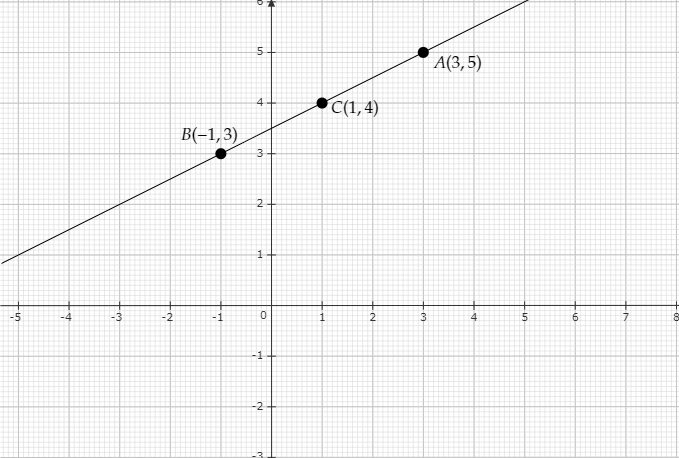# Plot the points $(3, 5)$ and $(-1, 3)$ on a graph paper and verify that the straight line passing through these points also passes through the point $(1, 4)$.

To do:

We have to plot the points $(3, 5)$ and $(-1, 3)$ on a graph paper and verify that the straight line passing through these points also passes through the point $(1, 4)$.

Solution:

 $x$ $3$ $-1$ $y$ $5$ $3$

Plot the points $A(3, 5)$ and $B(-1, 3)$ on the graph and join them to get the graph.

The above situation can be plotted graphically as below:We can observe that $(1, 4)$ lies on the straight line passing through the given points.